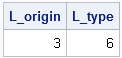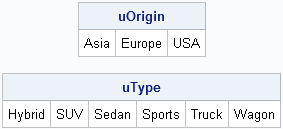More than a month ago I wrote a first article in response to an interesting article by Charlie H. titled Top 10 most powerful functions for PROC SQL. In that article I described SAS/IML equivalents to the MONOTONIC, COUNT, N, FREQ, and NMISS Functions in PROC SQL.

In this article, I discuss the UNIQUE functions in PROC SQL and in PROC IML.

The UNIQUE function in SQL finds the unique values of a variable. For categorical variables, it gives the levels of the variable. Charlie H. wrote a program that uses PROC SQL to count the number of levels for the Origin and Type variables in the SASHELP.CARS data set:

```proc sql; title'Unique() -- Find levels of categorical variables'; select count(unique(Origin)) as L_origin, count(unique(Type)) as L_type from sashelp.cars; quit;```The SAS/IML language also has a UNIQUE function. The UNIQUE function always returns a row vector that contains the unique sorted values of its argument, as shown in the following statements:

```proc iml; use sashelp.cars; read all var {Origin Type}; close sashelp.cars;   uOrigin = unique(Origin); uType = unique(Type); print uOrigin, uType;```Because you know that the output is a row vector, you can count the number of columns by using the NCOL function, as shown below:

```L_Origin = ncol(uOrigin); /** = 3 **/ L_Type = ncol(uType); /** = 6 **/```

I think that the UNIQUE function is one of the most important functions in SAS/IML because it enables you to compute statistics for each level of a categorical variable. Furthermore, the UNIQUE/LOC technique (which is described on p. 69 of my book, Statistical Programming with SAS/IML Software) is a highly useful technique that should be a part of every statistical programmer's toolbox.

ShareDistinguished Researcher in Computational Statistics

Rick Wicklin, PhD, is a distinguished researcher in computational statistics at SAS and is a principal developer of SAS/IML software. His areas of expertise include computational statistics, simulation, statistical graphics, and modern methods in statistical data analysis. Rick is author of the books Statistical Programming with SAS/IML Software and Simulating Data with SAS.

### 1 Comment

1.Hi, Rick!